In this article, we will find something out about the rotated array and how to find its minimum element.

Let’s get started.

## Given problem

Assuming that we have the sorted array that is described in the below image: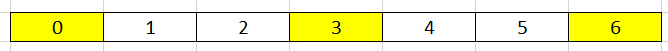After shifting this sorted array by some steps, we can have: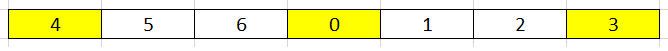The above array is called as a rotated array.

## Some properties of a rotated array

• When we divide a rotated array into two halves, at least one of the two halves will always sorted.

To understand this property, we can see a below image: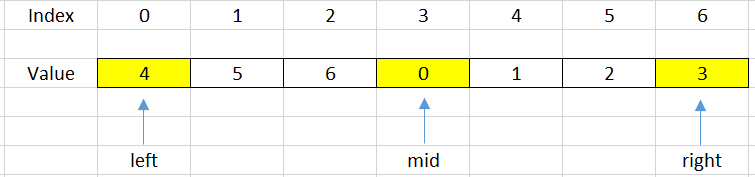If we choose the mid = 3 as the pivot point, we can find that our array is divided into two halves that are increased arrays.

–> So we can apply Binary Search for problems that are relevant to rotated array.

## Find minimum element of the rotated array

To solve this problem, we can have two solutions:

• Linear Search

The simple solution to solve it is to use Linear Search. We can scan all elements and compare them with the minimum element.

• Binary Search

Because the a part of array is sorted, so we can use Binary Search to solve it.

``````public static int findMinElement(int[] arr) {
int minPos = 0;
for (int i = 1; i < arr.length - 1; ++i) {
if (arr[minPos] > arr[i]) {
minPos = i;
}
}

return minPos;
}
``````

The complexity of Linear Search:

• Time complexity: O(n) - n is the number of elements of this array
• Space complexity: O(1)

## Using Binary Search to optimize solution

In a rotated array, we will have some cases that we will cope with when shift it.

1. Case 1 - when sorted array is not rotated with any steps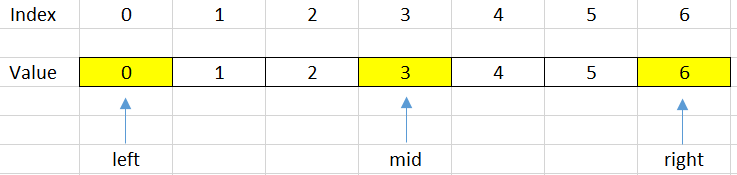In this case, our array is a sorted array but does not rotate with any steps. So, to check this case, we do the below expression:

`````` if (arr[left] <= arr[high]) {
return left;
}
``````
2. Case 2 - When our mid index points to the minimum elementSo, we will check it by using the following condition:

`````` int next = (mid + 1) % n;   // n is the length of the array
int prev = (mid + n - 1) % n;

if (arr[mid] < arr[next] && arr[mid] < arr[prev]) {
return mid;
}
``````
3. Case 3 - When our mid index points to the element that is belong to the sub-array. It does not contains the minimum element.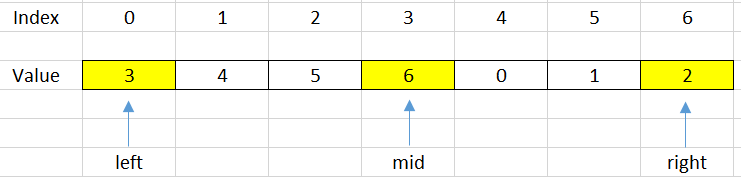In order to know exactly how we are under this case, we will use the below condition:

`````` if (arr[left] <= arr[mid]) {
left = mid + 1;
}
``````

Due to the minimum element that is belong to the other side, so we will shift the right side.

4. Case 4 - When our mid index points to the element that is belong to the sub-array. It contains the minimum element.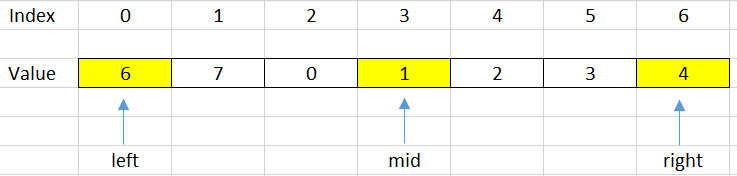`````` if (arr[mid] <= arr[right]) {
right = mid - 1;
}
``````

Based on the four conditions, we will have source code for this problem:

``````public static int findMinElement(int[] arr) {
int len = arr.length;
int left = 0;
int right = len - 1;

while (left <= right) {
if (arr[left] <= arr[right]) {  // Case 1: sorted array
return left;
}

int mid = left + (right - left) / 2;
int next = (mid + 1) % len;
int prev = (mid + len - 1) % len;
if (arr[mid] <= arr[next] && arr[mid] <= arr[prev]) {   // Case 2: mid index points to the minimum element
return mid;
} else if (arr[mid] <= arr[right]) {    // Case 4
right = mid - 1;
} else if (arr[mid] >= arr[left]) {     // Case 3
left = mid + 1;
}
}

return -1;
}
``````

The complexity of Binary Search:

• Time complexity: O(log(n)) - n is the number of elements of this array
• Space complexity: O(1)

## Wrapping up

• Understanding about what the rotated array is, and its properties.

• When we have two different trends in our array, we can use Binary Search to solve this problem.

• From this problem, we can find that in a rotated array, the index of the minimum element will be equal to the number of rotation of its array.